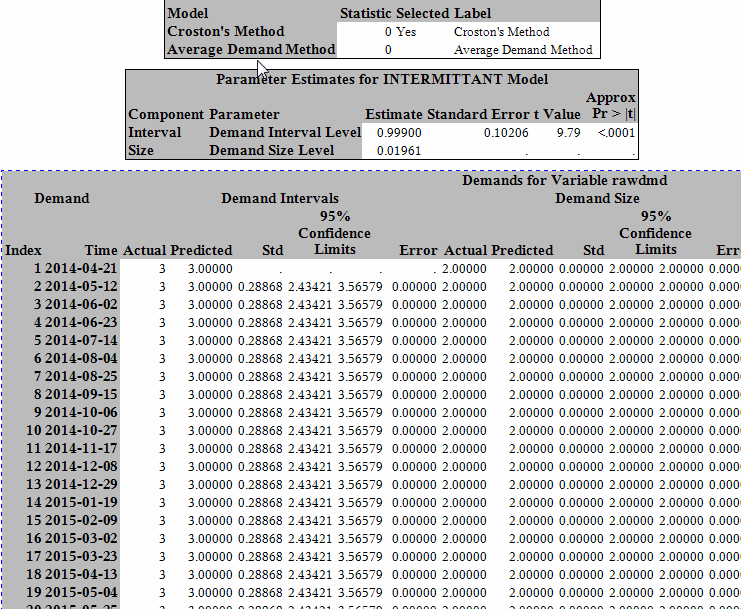## HPF IDM Demand Interval Prediction Data Export

I am working on a forecast value add project where I plan to compare SAS forecasts for multiple SKUs to our current forecasting system. To do this, I will need to export forecasts for each SKU into a data set that I can compare against our current forecasts.  This works fine for SKUs with continuous demand, but in order to compare the forecasts for intermittent demand I will need forecasts for both the Average Demand Size and the Demand Interval for these SKUs.  I can export these by SKU, however I'd like to design a data set of forecasts for multiple SKUs.  How do I produce the Demand Interval forecasts using the data sets exported from HPF?  I can see parameters in OUTEST, but I do not know how to fit those together to get a number.

I have been using the following code and I am attaching an image to display the ODS output I receive that shows me the interval estimate.

The TL/DR version is: How does ODS calculate the Demand Interval Prediction?

`````` * assign project settings ;
%let HPF_SETMISSING=0;
%let HPF_ZEROMISS=NONE;
%let ODSSTYLE=STATISTICAL;
%let HPF_TRIMMISS=BOTH;
%let HPF_BACK=0;
%let HPF_START='07Apr2014'd;
%let HPF_START_ENABLED=0;
%let HPF_END='14Mar2016'd;
%let HPF_END_ENABLED=0;
%let HPF_HORIZON_START='21Mar2016'd;
%let HPF_HORIZON_START_ENABLED=0;
%let HPF_SELECT_MINOBS_TREND=2;
%let HPF_SELECT_MINOBS_SEASONAL=2;
%let HPF_SELECT_MINOBS_NON_MEAN=2;
%let HPF_DIAGNOSE_INTERMITTENT=2.0;
%let HPF_SELECT_HOLDOUT=4;
%let HPF_SELECT_HOLDOUTPCT=50;
%let HPF_SELECT_ENDZEROS_MAXNUM=5;
%let HPF_SELECT_ENDZEROS_MAXPCT=50;
%let HPF_SELECT_ENDZEROS_MINOBS=1;
%let HPF_SELECT_CRITERION=MAE;
%let HPF_COMPONENTS=INTEGRATE;
%let HPF_FORECAST_ALPHA=0.05;
%let HPF_RECONCILE_WEIGHTED=;
%let HPF_RECONCILE_IGNOREMISSF=;
%let HPF_RECONCILE_LOCKZERO=;
%let HPF_RESTRICT_OVRD_RECON_DIR=0;
%let HPF_FORCE_OVRD_CONFLICT_CHECK=1;
%let HPF_DO_NOT_ALLOW_NEG_FORECAST=;
%let HPF_CREATE_INDEX_FILES=;
%let HPF_CREATE_OUTCOMPONENT_DATA_SET=;
%let HPF_DIAGNOSE_ARIMAX=;
%let HPF_DIAGNOSE_ESM=;
%let HPF_RETAINCHOOSE=RETAINCHOOSE;
%let HPF_SELECTION_LIST=FSDEMO.CUST_REPO.TOP_2;
%let HPF_OUTLIER_FILTER=SUBSET;
%let HPF_COMBINE=;
%let HPF_COMBINE_CRITERION=RMSE;
%let HPF_COMBINE_MISSMODE=MISSING;
%let HPF_COMBINE_METHOD=AVERAGE;
%let HPF_COMBINE_STDERR=DIAG;
%let HPF_ARIMA_IDENTIFY=BOTH;
%let HPF_USE_DIAGNOSE=;
%let HPF_TRANSTYPE=NONE;
%let HPF_TRANSOPT=MEDIAN;
proc hpfengine data=_leaf.DATA (where= (corp_item_num=4756656.0 or corp_item_num= 2397586.0))
inest=_leaf.outest
seasonality=52
errorcontrol=(severity=ALL, stage=(PROCEDURELEVEL DATAPREP SELECTION ESTIMATION FORECASTING))
EXCEPTIONS=CATCH modelrepository=work.TemLevModRep_leaf
print=(FORECASTS select estimates)
task = select( alpha=&HPF_FORECAST_ALPHA criterion=&HPF_SELECT_CRITERION endzeros=(maxnum=&HPF_SELECT_ENDZEROS_MAXNUM) endzeros=(maxpct=&HPF_SELECT_ENDZEROS_MAXPCT) endzeros=(minobs=&HPF_SELECT_ENDZEROS_MINOBS) holdout=&HPF_SELECT_HOLDOUT holdoutpct=&HPF_SELECT_HOLDOUTPCT minobs=&HPF_SELECT_MINOBS_NON_MEAN  minobs=(season=&HPF_SELECT_MINOBS_SEASONAL) minobs=(trend=&HPF_SELECT_MINOBS_TREND)

seasontest=none intermittent=&HPF_DIAGNOSE_INTERMITTENT override)
out=work.out outfor=work.outfor outstat=work.outstat
outest=work.outest
outstatselect=work.outstatselect
outmodelinfo=work.outmodelinfo
scorerepository=work.scorerepository outsum=work.outsum
outcomponent=work.outcomponent
inevent=_project.EventRepository;
by client corp_item_num ;
score;
id week_of interval=WEEK.2 format=YYMMDD10. acc=total notsorted horizonstart=&HPF_HORIZON_START start=&HPF_START;
forecast rawdmd /
setmissing=&HPF_SETMISSING trimmiss=&HPF_TRIMMISS zeromiss=&HPF_ZEROMISS
;

run;``````

I'm using SAS 9.3 and EG 5.1.  This is all using Forecast Server 12.1.1 ACCEPTED SOLUTION

Accepted Solutions

## Re: HPF IDM Demand Interval Prediction Data Export

The interval and size forecasts are stored in the ODS table called Demands. You can "capture" the ODS table using ODS OUTPUT Demands = idm_demand_predictions; and change the PROC HPFENGINE option PRINT= option to PRINT = ALL. If you don't want all the ODS ouptuts printed in the listing/html output, you can use the following trick before and after the proc call to capture just the Demand ODS table:

ods exclude all;
ods output demands = idm_demand_predictions;

proc hpfengine ...... print = all;
......
run;
ods exclude none;

2 REPLIES 2

## Re: HPF IDM Demand Interval Prediction Data Export

The interval and size forecasts are stored in the ODS table called Demands. You can "capture" the ODS table using ODS OUTPUT Demands = idm_demand_predictions; and change the PROC HPFENGINE option PRINT= option to PRINT = ALL. If you don't want all the ODS ouptuts printed in the listing/html output, you can use the following trick before and after the proc call to capture just the Demand ODS table:

ods exclude all;
ods output demands = idm_demand_predictions;

proc hpfengine ...... print = all;
......
run;
ods exclude none;

## Re: HPF IDM Demand Interval Prediction Data Export

Thank you so much! This gives me just what I was looking for.
Discussion stats
• 2 replies
• 904 views
• 0 likes
• 2 in conversation